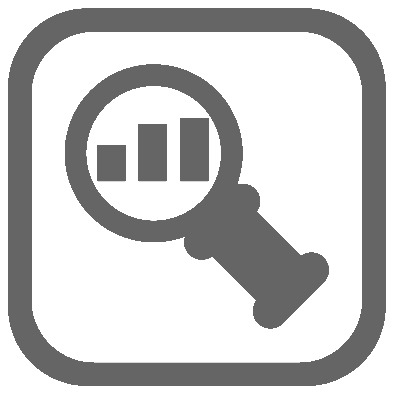## LIS Analysis - in depth analysis of point clouds

This package contains tools for the in-depth analysis of point cloud data like 3D distance measurement between two point clouds, positional accuracy estimation, the calculation of various point features by neighborhood analysis (including height features, density features, geometric features, local plane features and Eigenvalue features), the calculation of segment features for object-based classification, statistical analyses and fuzzy c-means clustering.

Tool: Distances Between Point Clouds

Features
• 3D distance measurement between two point clouds
• measurement along normal vector direction between two fitted planes
• choice of analysis scale and thus consideration of surface roughness
• automatic derivation of a level-of-detection (LOD) based on surface roughness, point count, positional (range, laser beam incidence angle) and registration error
• output of measured 3D distance, displacement vector, LOD, etc.
Application
• real 3D distance measurement between two point clouds
• change detection and quantification
• error assessment
• quality control

Tool: Feature Statistics (PC)

Features
• calculation of cumulative statistics of pre-computed point cloud attributes: count, minimum, maximum, range, sum, mean, standard deviation, median and majority
• in the case a segment identifier is provided the statistics are calculated per segment
• calculation results can be attached to the point cloud or reported in a table
Application
• statistical analysis of (pre-computed) point attributes / features

Tool: Fuzzy C-Means Clustering

Features
• Fuzzy C-Means clustering on point cloud attributes
• application of a minimum degree of membership
• optional output of derived cluster centers
Application
• cluster analysis
• unsupervised classification

Tool: Point Cloud Accuracy Estimation

Features
• estimation of positional accuracy of each point based on scanner position, beam divergence and local surface orientation
• calculation of range (distance to scanner), footprint diameter, incidence angle, horizontal error, vertical error and total error
Application
• estimation of positional uncertainty of each point in a point cloud
• calculation of range (e.g. for intensity normalization)

Tool: Point Cloud Attribute Histogram

Features
• calculation of histograms of point cloud attributes (reported in a table)
• user-defined bin size and bin minimum
Application
• determination of point cloud attribute value distributions

Tool: Point Cloud Features

Features
• calculation of geometrical and echo-based point features based on the analysis of the local point neighborhood
• height features: 2D height difference, 2D height variance, 2D elevation range, 3D elevation range, 3D height variance, offset to a raster dataset
• density features: 2D point density, 3D point density, 3D/2D point density ratio, 3D/2D neighbor ratio, 3D/2D slope-adaptive echo ratio
• local plane features: normal vector, slope, aspect, distance, deviation, standard deviation, quality of fit
• Eigenvalue features: Eigenvalues, Eigenvectors, anisotropy, omnivariance, planarity, linearity, scatter, entropy, Sneed-Folk-Form-Index, vertical angle of largest Eigenvalue, vertical angle of smallest Eigenvalue, horizontal angle of largest Eigenvalue, boundary angle, geometric curvature, change of curvature
• radius and k-nearest neighbor search
• least-squares and robust plane fitting
• optional: use of core points and a separate neighborhood point cloud, e.g. to perform the analysis on different scales
Application
• calculation of a manifold set of point features describing the local point neighborhood
• point cloud (statistical) analysis
• point cloud classification

Tool: Point Neighborhood Statistics

Features
• calculation of cumulative statistics of point cloud attributes from the point's neighborhood: count, minimum, maximum, range, mean, median, standard deviation, percentile and skewness
• 2D or 3D neighborhood
• optional: use of core points and a separate neighborhood point cloud, e.g. to perform the analysis on different scales
Application
• computation of statistical measures from the local point neighborhood
• computation of statistical measures from the neighborhood of separate point cloud

Tool: Segment Features

Features
• calculation of features for point cloud segments
• analysis of all points belonging to a (pre-computed) segment instead of using a search radius
• geometric features: point count, length 2D/3D, width 2D/3D, height 2D/3D, shape ratio 2D/3D, bounding box area 2D/3D and bounding box volume 2D/3D
• polygon features: polygon area 2D/3D, perimeter 2D/3D, P/A 2D/3D, Deqpc 2D/3D, sphericity 2D/3D, shape index 2D/3D
• plane features: normal vector, slope, aspect, distance, deviation, standard deviation
• Eigenvalue features: Eigenvalues, Eigenvectors, anisotropy, omnivariance, planarity, linearity, scatter, entropy, Sneed-Folk-Form-Index, vertical angle, horizontal angle
Application
• calculation of a manifold set of segment features describing the segment properties
• (statistical) analysis of segments
• object-based point cloud classification